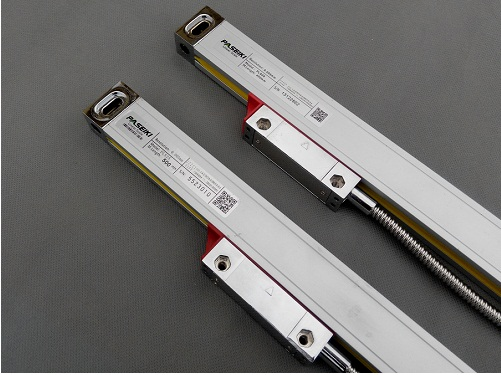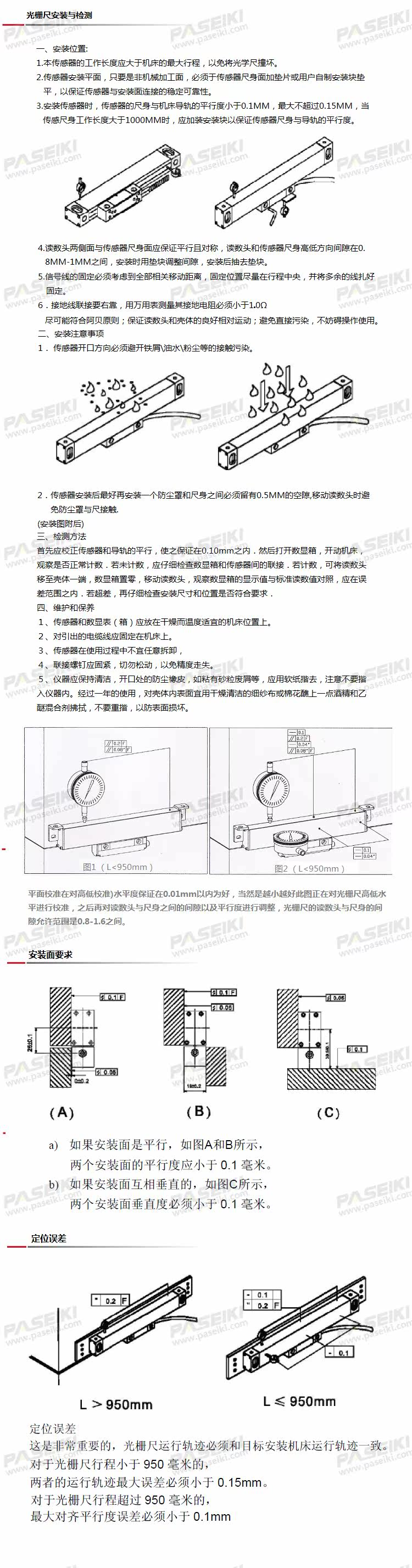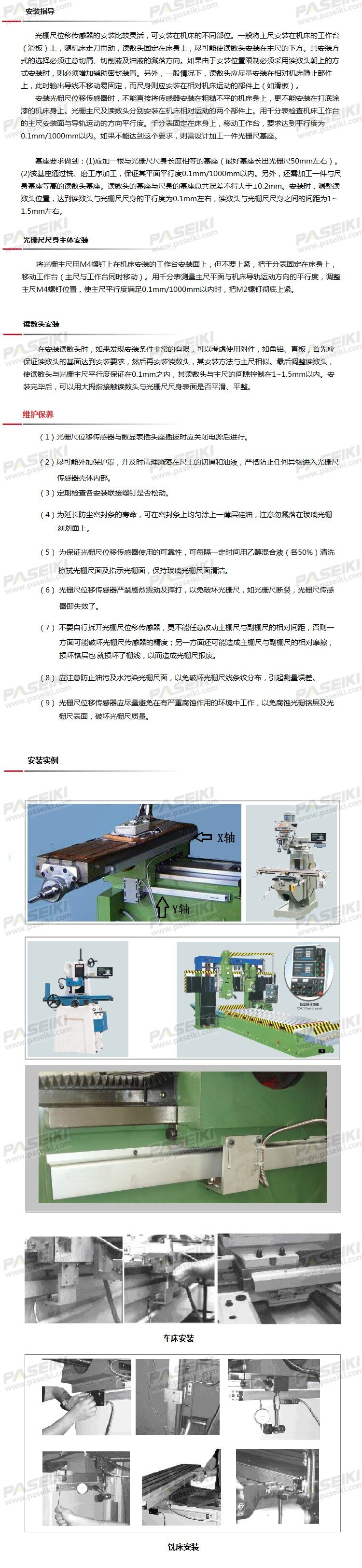### 通用型光栅尺

• 品牌   PASEIKI
• 型号   PLS25/21
• 描述

PLSEIKI光栅尺按外观大小分为： 通用光栅尺-PLS2系列（普通型）（普通机床用、PLC用） 小型光栅尺-PLS1系列（紧凑型）（有安装位置限制的场合使用） 大型光栅尺-PLS3系列（紧固型...

PLSEIKI光栅尺按外观大小分为：PLS2系列

PLS3系列

>> PLS系列光栅尺输出信号及接线图【双层防护胶封设计】

【每支尺均通过激光校验】

>> 订购指南

 类别及量程 尺身型号 分辨率 零位 电源电压 输出方式 数据线长度 接头方式 PLS 2 5 C 5 L MST DB9 1=小型尺身 1=1μm N=无零位 5 = 5V L=线性输出 M01N=1m SC=无接头 2=通用尺身 5=5μm C=中间零位 24 = 24V P=PNP输出 M02/N=2m DB9=DB9 3=加固尺身 L=左零位 0528 =5~28V N=NPN输出 M10/N=10m 2P=PLC尺身 R=右零位 MST=标准线长